# Azure 数据资源管理器中的时序分析

Azure 数据资源管理器 (ADX) 持续从云服务或 IoT 设备收集遥测数据。 分析这些数据可获得各种见解，例如，监视服务的运行状况、物理生产流程和使用趋势。 分析是针对所选指标的时序执行的，以找出某种模式与其典型基线模式之间的偏差。 ADX 原生支持创建、操作和分析多个时序。 本主题介绍如何使用 Azure 数据资源管理器在几秒钟内创建和分析数千个时序，实现准实时的监视解决方案和工作流。

## 时序的创建

[单击以运行查询]

``````demo_make_series1 | take 10
``````

2016-08-25 09:12:35.4020000 Chrome 51.0 Windows 7 英国
2016-08-25 09:12:41.1120000 Chrome 52.0 Windows 10
2016-08-25 09:12:46.2300000 Chrome 52.0 Windows 7 英国
2016-08-25 09:12:46.5100000 Chrome 52.0 Windows 10 英国
2016-08-25 09:12:46.5570000 Chrome 52.0 Windows 10 立陶宛共和国
2016-08-25 09:12:47.0470000 Chrome 52.0 Windows 8.1 印度
2016-08-25 09:12:51.3600000 Chrome 52.0 Windows 10 英国
2016-08-25 09:12:51.6930000 Chrome 52.0 Windows 7 荷兰
2016-08-25 09:12:56.4240000 Chrome 52.0 Windows 10 英国
2016-08-25 09:13:08.7230000 Chrome 52.0 Windows 10 印度

[单击以运行查询]

``````let min_t = toscalar(demo_make_series1 | summarize min(TimeStamp));
let max_t = toscalar(demo_make_series1 | summarize max(TimeStamp));
demo_make_series1
| make-series num=count() default=0 on TimeStamp from min_t to max_t step 1h by OsVer
| render timechart
``````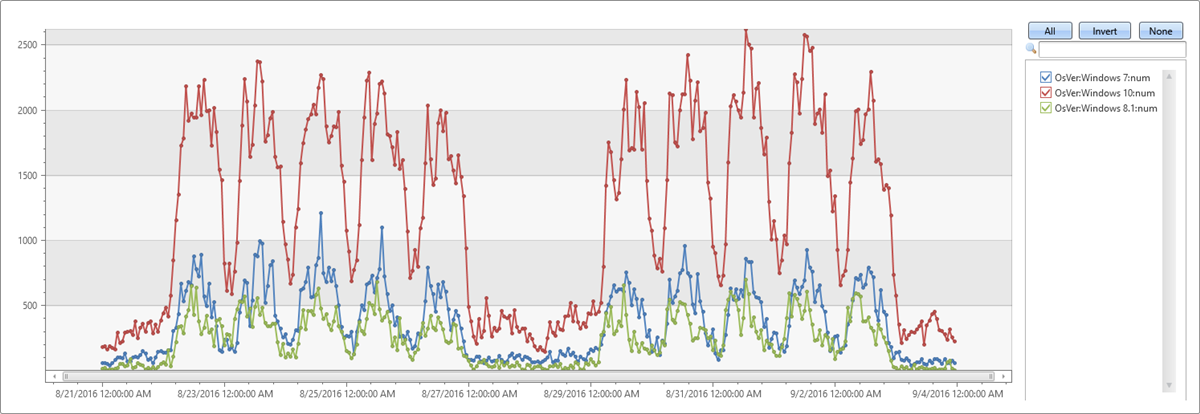## 时序分析函数

### 筛选

• 有两个泛型筛选函数：
• 通过将大小为 5 个箱的新移动平均时序（名为 ma_num）添加到查询，来 `Extend`（扩展）时序集：

[单击以运行查询]

``````let min_t = toscalar(demo_make_series1 | summarize min(TimeStamp));
let max_t = toscalar(demo_make_series1 | summarize max(TimeStamp));
demo_make_series1
| make-series num=count() default=0 on TimeStamp in range(min_t, max_t, 1h) by OsVer
| extend ma_num=series_fir(num, repeat(1, 5), true, true)
| render timechart
``````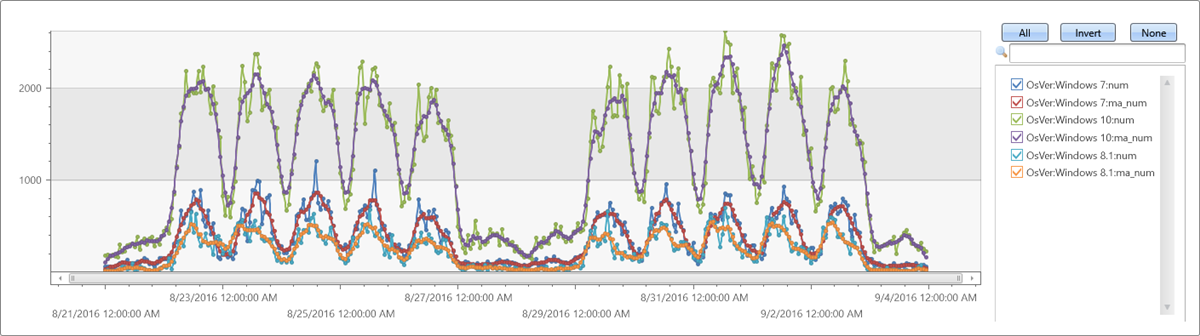### 回归分析

• 使用 series_fit_line() 将最佳线条拟合到时序即可实现一般趋势检测。
• 使用 series_fit_2lines() 可以检测相对于基线的趋势变化，这种变化在监视方案中非常有用。

[单击以运行查询]

``````demo_series2
| extend series_fit_2lines(y), series_fit_line(y)
| render linechart with(xcolumn=x)
``````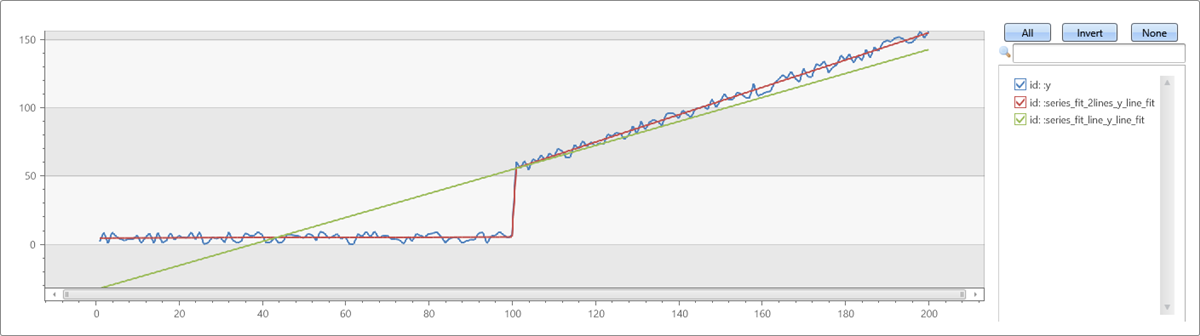• 蓝色：原始时序
• 绿色：拟合的线条
• 红色：两条拟合的线条

### 季节性检测

[单击以运行查询]

``````demo_series3
| render timechart
``````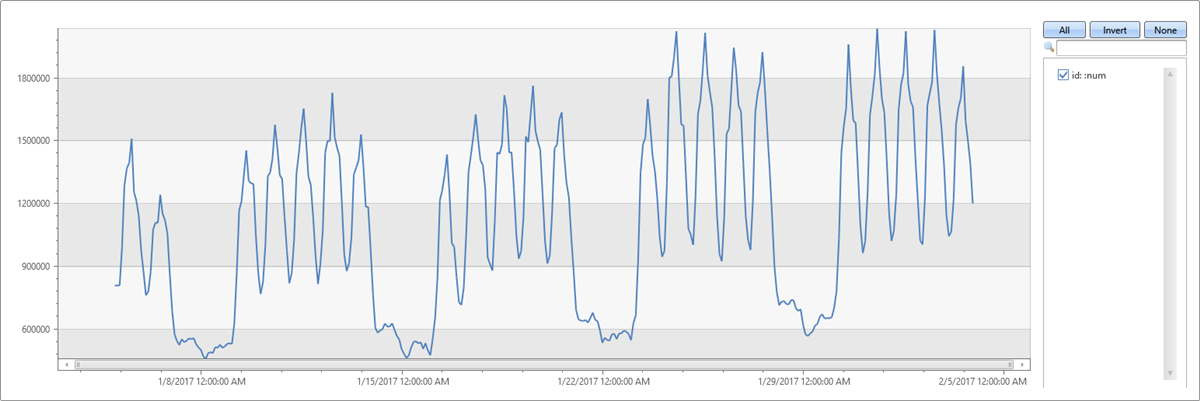[单击以运行查询]

``````demo_series3
| project (periods, scores) = series_periods_detect(num, 0., 14d/2h, 2) //to detect the periods in the time series
| mv-expand periods, scores
| extend days=2h*todouble(periods)/1d
``````
periods 评分 days
84 0.820622786055595 7
12 0.764601405803502 1

### 元素对应的函数

[单击以运行查询]

``````let min_t = toscalar(demo_make_series1 | summarize min(TimeStamp));
let max_t = toscalar(demo_make_series1 | summarize max(TimeStamp));
demo_make_series1
| make-series num=count() default=0 on TimeStamp in range(min_t, max_t, 1h) by OsVer
| extend ma_num=series_fir(num, repeat(1, 5), true, true)
| extend residual_num=series_subtract(num, ma_num) //to calculate residual time series
| where OsVer == "Windows 10"   // filter on Win 10 to visualize a cleaner chart
| render timechart
``````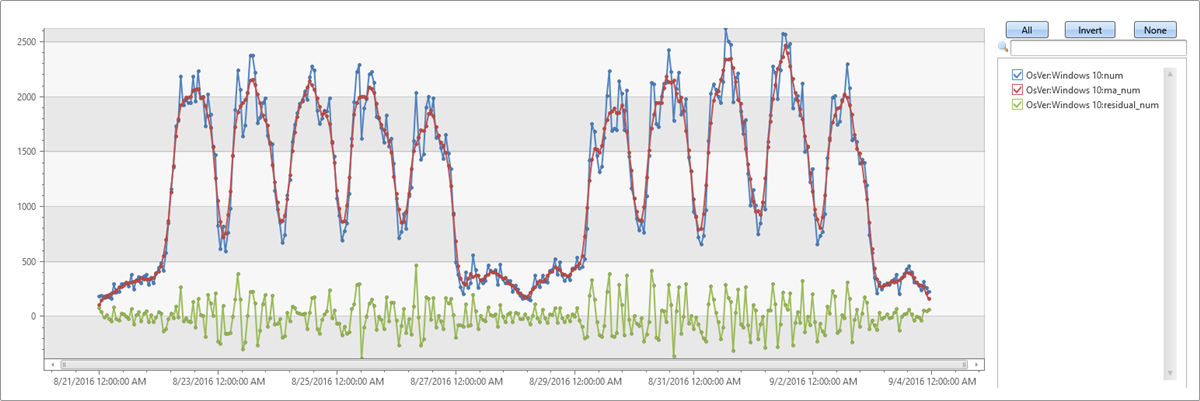• 蓝色：原始时序
• 红色：经过平滑处理的时序
• 绿色：剩余的时序

## 时序的大规模工作流

[单击以运行查询]

``````demo_many_series1
| take 4
``````
2016-09-11 21:00:00.0000000 Loc 9 5117853934049630089 262 0
2016-09-11 21:00:00.0000000 Loc 9 5117853934049630089 241 0
2016-09-11 21:00:00.0000000 Loc 9 -865998331941149874 262 279862
2016-09-11 21:00:00.0000000 Loc 9 371921734563783410 255 0

[单击以运行查询]

``````demo_many_series1
| summarize num=count(), min_t=min(TIMESTAMP), max_t=max(TIMESTAMP)
``````
num min_t max_t
2177472 2016-09-08 00:00:00.0000000 2016-09-11 23:00:00.0000000

[单击以运行查询]

``````let min_t = toscalar(demo_many_series1 | summarize min(TIMESTAMP));
let max_t = toscalar(demo_many_series1 | summarize max(TIMESTAMP));
demo_many_series1
| render timechart with(ymin=0)
``````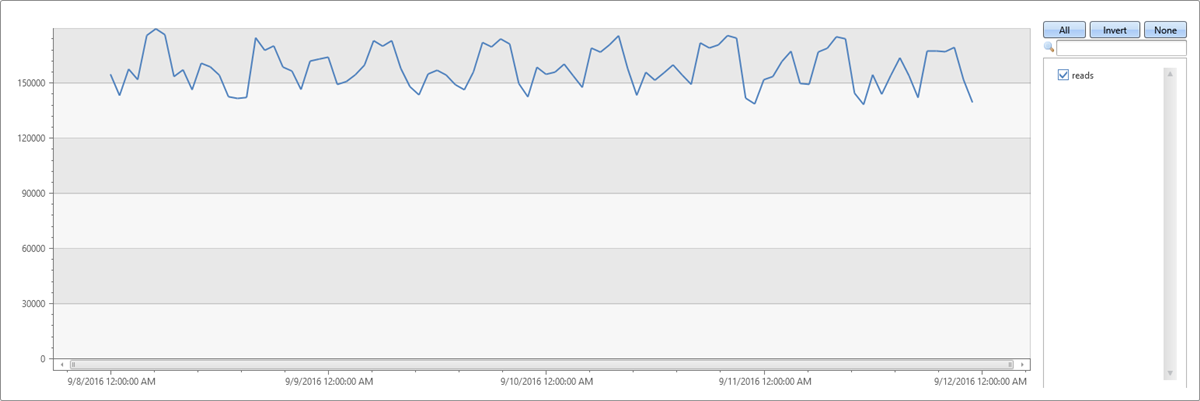[单击以运行查询]

``````demo_many_series1
| summarize by Loc, Op, DB
| count
``````

18339

[单击以运行查询]

``````let min_t = toscalar(demo_many_series1 | summarize min(TIMESTAMP));
let max_t = toscalar(demo_many_series1 | summarize max(TIMESTAMP));
demo_many_series1
| make-series reads=avg(DataRead) on TIMESTAMP in range(min_t, max_t, 1h) by Loc, Op, DB
| extend (rsquare, slope) = series_fit_line(reads)
| top 2 by slope asc
| render timechart with(title='Service Traffic Outage for 2 instances (out of 18339)')
``````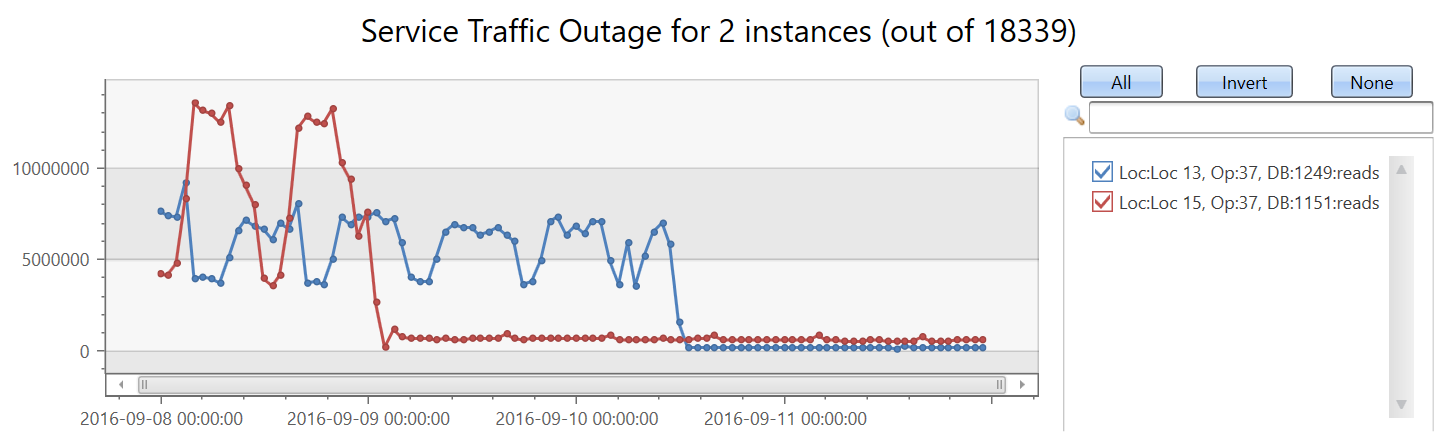[单击以运行查询]

``````let min_t = toscalar(demo_many_series1 | summarize min(TIMESTAMP));
let max_t = toscalar(demo_many_series1 | summarize max(TIMESTAMP));
demo_many_series1
| make-series reads=avg(DataRead) on TIMESTAMP in range(min_t, max_t, 1h) by Loc, Op, DB
| extend (rsquare, slope) = series_fit_line(reads)
| top 2 by slope asc
| project Loc, Op, DB, slope
``````
Loc Op DB slope
Loc 15 37 1151 -102743.910227889
Loc 13 37 1249 -86303.2334644601